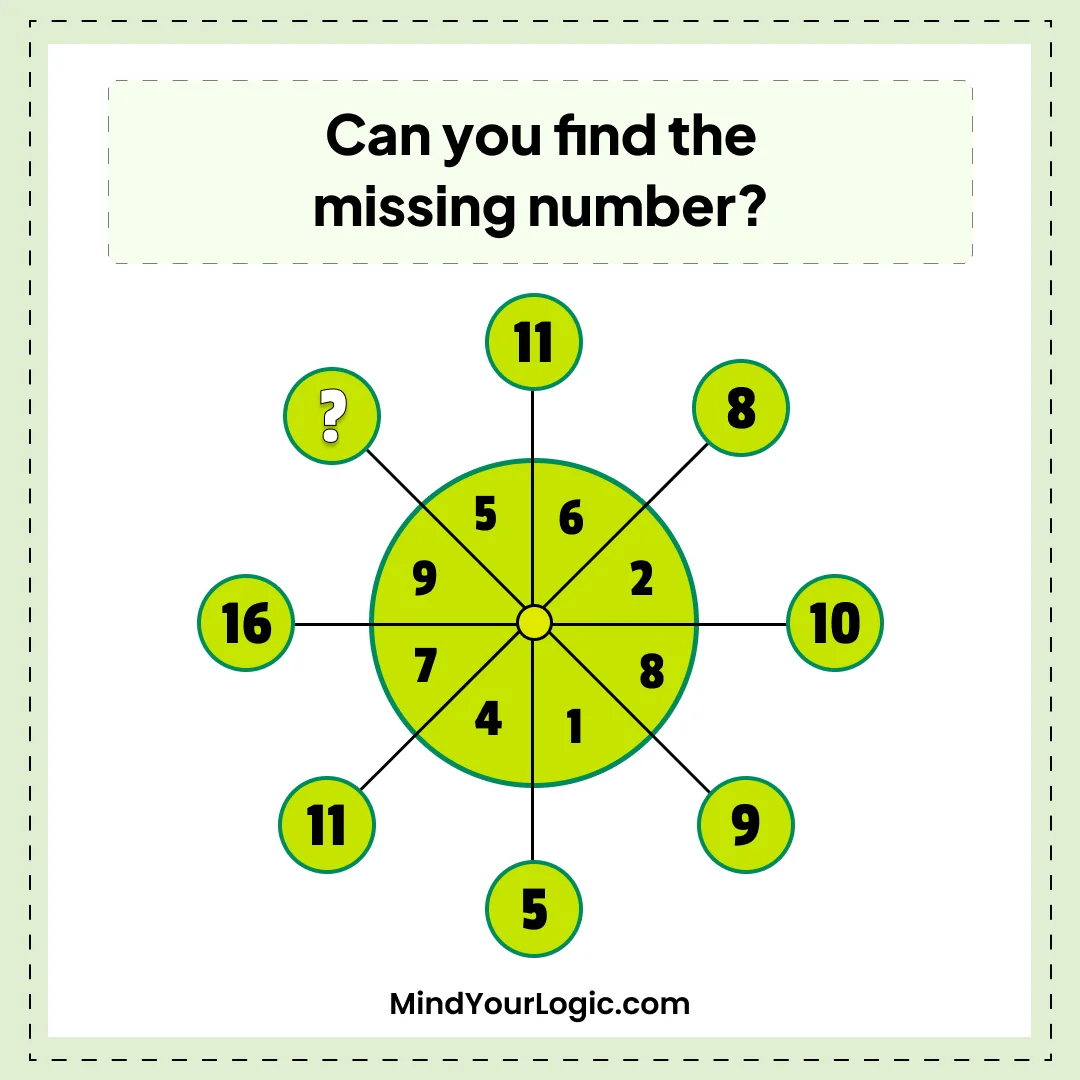# Find the value - Math Riddle

###### 74.Math Riddles
`Can you find the value of the missing number?`• A. 14
• B. 9
• C. 11
• D. 16
• ```Explanation :

The outer number is the sum of the numbers in the two segments immediately across the line connecting big Circle to small Circle.

i.e.
5+6=11,
6+2=8,
2+8=10,
8+1=9,
1+4=5,
4+7=11,
7+9=16
therefore,
9+5 =14```